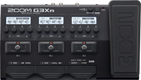Discussion in 'Zoom G3n/G3Xn/G5n' started by Rebekah, Feb 28, 2021.Device: Zoom G3Xn
Firmware: 2.20

Name on device: Deep Fried
Optimized for: Guitar Amp

Effects chain:This is my take on Marty Friedman's tone on the track Holy Wars. Hope you like it!

Effect: "ZNR" (Dynamics), active - "yes"
"Detect" = GTRIN
"Depth" = 60
"Threshold" = 30
"Decay" = 0

Effect: "TS Drive" (Overdrive / Distortion), active - "yes"
"Gain" = 100
"Boost" = On
"Tone" = 100
"Volume" = 43

Effect: "HG THRTTL" (Overdrive / Distortion), active - "yes"
"Gain" = 100
"Tone" = 100
"MdCut" = 43
"Volume" = 82

Effect: "Gt GEQ 7" (Filter), active - "yes"
"100 Hz" = 1.0
"200 Hz" = 1.0
"400 Hz" = -2.5
"800 Hz" = 2.5
"1.6 kHz" = -2.5
"3.2 kHz" = -6.0
"6.4 kHz" = 3.5
"Volume" = 85
"100 Hz" = 1.0
"200 Hz" = 1.0
"400 Hz" = -3.0
"800 Hz" = -4.5
"1.6 kHz" = -1.5
"3.2 kHz" = -7.0
"6.4 kHz" = 1.5
"Volume" = 94
"Parameter set" = B

Effect: "PDL Vol" (Pedal), active - "yes"
"Volume" = 100
"Min" = 0
"Max" = 100
"Curve" = A

Effect: "Delay" (Delay), active - "no"
"Time" = Quarter
"F.B." = 45
"Mix" = 40
"Tail" = On

Patch Volume: 100